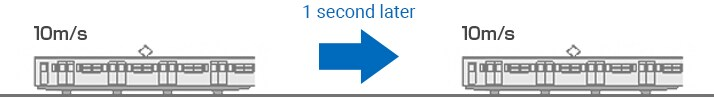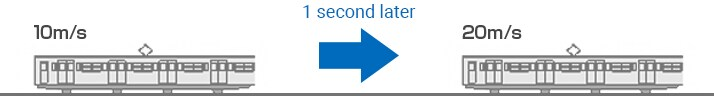# What Is Acceleration?

Acceleration sensors are designed to measure changes in speed over one second. Then, the magnitude of vibration is obtained from the acceleration. This section explains the basics of acceleration, including its concept and unit of measurement.

## What is acceleration?

“Acceleration” is a rate of change in speed per unit of time.Therefore, the acceleration is “0” when the speed does not change, as shown above.
Even if the speed is slow, an acceleration is generated if there are any changes in the speed.
In the SI unit system, the acceleration is expressed in m/s2.
This shows how much the speed (m/s) changes in one second.In the above figure, the acceleration is 10 m/s2 because the speed changes by 10 m/s in one second.

## Units of acceleration

The following three units are used in general.

Unit name Symbol Definition
Meter per second per second m/s2 SI unit system
Gravitational acceleration G The acceleration when an object naturally falls by the gravity of the earth
Gal Gal “1 Gal” means that the speed increases by 1 cm/s per second.

## Relationships between acceleration, speed, and displacement

The following correlations exist between acceleration, speed, and displacement.Integration of the acceleration gives the speed.
Integration of the speed gives the displacement.
Differentiation of the displacement gives the speed.
Differentiation of the speed gives the acceleration.

INDEX